# The Most Important Data Science Tool for Market and Customer Segmentation

### Use K-means and let AI advise you how many segments there (really) are.Image Credits: unsplash.com

Market and customer segmentation are some of the most important tasks in any company. The segmentation done will influence marketing and sales decisions, and potentially the survival of a company.

Surprisingly, despite the advances in machine learning, few marketers are using such technologies to augment their all-important market and customer segmentation efforts.

In this article, I will show you how to augment your segmentation analysis with a simple, yet powerful machine learning technique called K-means. Learning this will give you an edge over your competitors (and colleagues).

## So what’s K-means?

K-means is a popular clustering algorithm for unsupervised machine learning. It groups similar data points into a predefined number of groups.

Let me explain each term for you:

• Clustering: a machine learning technique for identifying and grouping similar data points (e.g. customers) together.
• Unsupervised machine learning: you don’t need to provide labelled data to the algorithm on how to group the customers. It will scan through all information associated with each customer and learn the best way to group them together.
• A predefined number of groups: you need to tell K-means how many groups to form. This is the only input needed from you.

Here is an analogy to the above concepts: Imagine you have some toys and without providing further instruction, you ask your kid to separate the toys into three groups. Your kid will play around and eventually find his own best way to form three groups of similar toys.Image Credits: unsplash.com

## OK … so how does K-means work?

Let’s assume that you think there are 3 potential segments of customers.

K-means will initiate 3 points (i.e. centroids) at random locations and slowly fit each data point to the nearest centroid. Each data point represents one customer, and the customer closest to the same centroid will be in the same group.

The centroids’ locations are adjusted automatically based on the last nearest customer allocated to them. Doing so, it will learn on its own to find other customers with similar characteristics.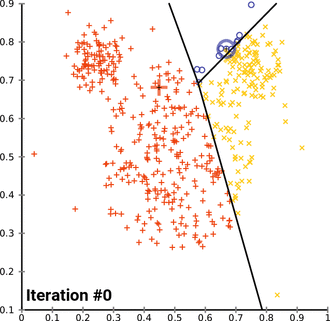K-means identifying 3 clusters in a data set. Source: Wikipedia

## What? That looks simple. I could do the grouping visually myself!

The 2-dimensional representation of customers above is a simplified form of visualising the data.

Each information associated with a customer represents one dimension of data. For instance, if you are just plotting the items and quantity purchased, then that’s 2-dimension. Once you consider additional information for each customer, such as country of residence and total spending, the complexity jumps to 4-dimension!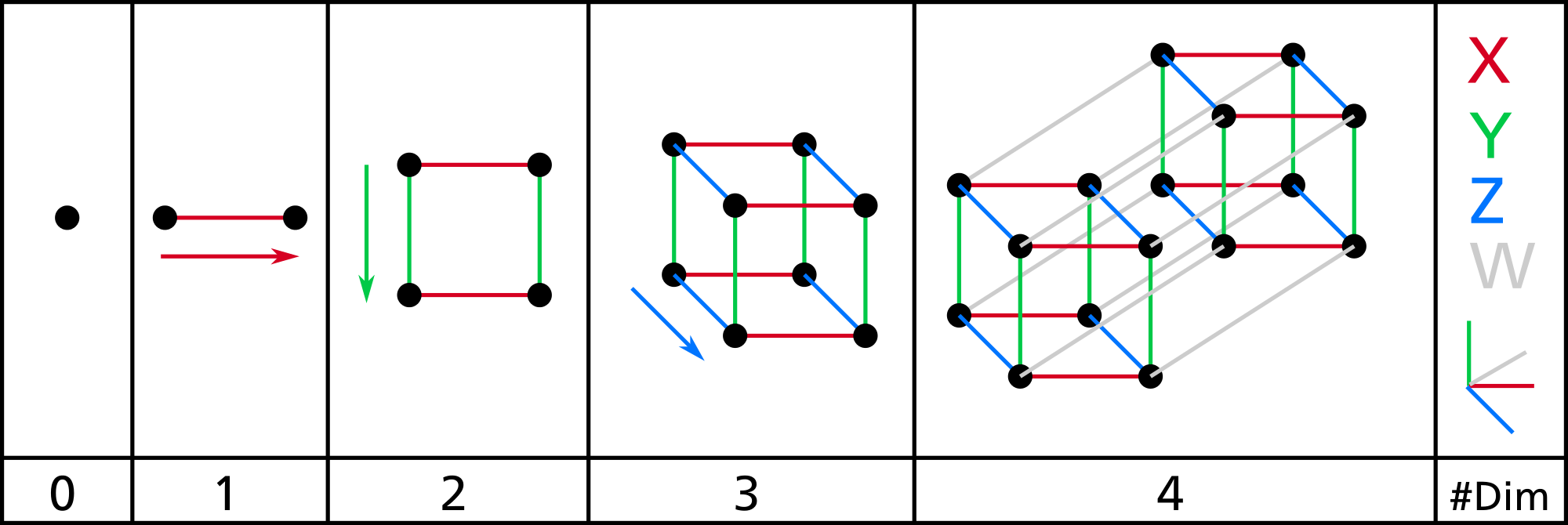Visualisation of different dimensions. Source: Wikipedia

It is hard for us to imagine grouping items together beyond 3-dimensional space, but not so for machine learning. This makes machine learning much more powerful than traditional methods in finding meaningful segments.

Machine learning can make sense of multiple dimensions beyond our imagination, find similar characteristics of customers based on their information, and group similar customers together.

That’s the beauty of it!

## But how do I know what’s the optimal number of groups to form?

You can find the optimal number of groups by following these two principles:

1. Customers in the same cluster should be close together (tight intra-cluster distance)
2. Each different cluster of customers should be far from each other (far inter-cluster distance)

Here’s another way of interpreting for the above principles:

1. Birds of the similar feather flock together. They flock close to each other to find like-minded friends; the more like-minded they are, the closer they flock together.
2. Different flocks do not come near each together. Each flock is proud of their unique identity; the more distinct their identity, the further they will distance themselves from other flocks.

One method for finding the optimal number of groups is to use Silhouette Score. It takes into consideration both the intra-cluster and inter-cluster distance and returns a score; the lower the score, the more meaningful the clusters formed.

One of the most challenging aspects of using K-means is deciding how many clusters to form. This can be identified mathematically by using Silhouette Score.

## Great. Could you illustrate using K-means to segment an actual customer dataset?

I will illustrate using K-means to perform RFM (Recency, Frequency, and Monetary) customer segmentation. The data source is from an actual online retailer in the UK.

I have already pre-processed the data by performing the following step:

1. Extract most recent 1-year transactions data.
2. Calculate the Recency of each customer by their latest transaction date.
3. Calculate the Frequency of each customer by summing the number of invoices tagged to each customer.
4. Calculate the Monetary Value of each customer by summing up their respective total spend.
``````# Calculate 1-year date range from latest data
end_date = df['Date'].max()

# Filter 1-year data range from original df
start_date = end_date - pd.to_timedelta(364, unit='d')
df_rfm = df[(df['Date'] >= start_date) & (df['Date'] <= end_date)]

# Create hypothetical snapshot date
snapshot_date = end_date + dt.timedelta(days=1)

# Calculate Recency, Frequency and Monetary value for each customer
df_rfm = df_rfm.groupby(['CustomerID']).agg({
'Date': lambda x: (snapshot_date - x.max()).days,
'InvoiceNo': 'count',
'TotalSum': 'sum'})

# Rename the columns
df_rfm.rename(columns={'Date': 'Recency',
'InvoiceNo': 'Frequency',
'TotalSum': 'MonetaryValue'}, inplace=True)

# Print top 5 rows
print(df_rfm.head())``````

Below is a snapshot of the RFM values of each customer that I created: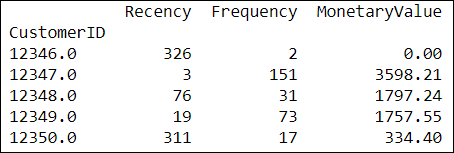RFM value of each customer.

## Anything else that I need to do before implementing K-means?

K-means gives the best result under the following conditions:

1. Data’s distribution is not skewed (i.e. long-tail distribution)
2. Data is standardised (i.e. mean of 0 and standard deviation of 1)

Why? Recall that K-means groups similar customers together based on their distance from centroids.

The location of each data point on the graph is determined by considering all information associated with the specific customer. If any of the information is not on the same distance scale, K-means might not form meaningful clusters for you.

Machine learning means learning from data. To get the best result, you should prepare the data to make it easy for the machine to learn.

Here are the exact steps to prepare the data before using K-means :

1. Plot distribution charts to check for skewness. If the data is skewed (i.e. has long-tail distribution), perform log transformation to reduce the skewness
2. Scale and centre the data to have a mean of 0 and variance of 1

I first check for skewness of data by plotting a distribution plot of Recency, Frequency, and MonetaryValue:Distribution Plots of RFM. All variables are heavily skewed.

I performed log transformations to reduce the skewness of each variable. Below is the distribution plots of RFM after log transformation: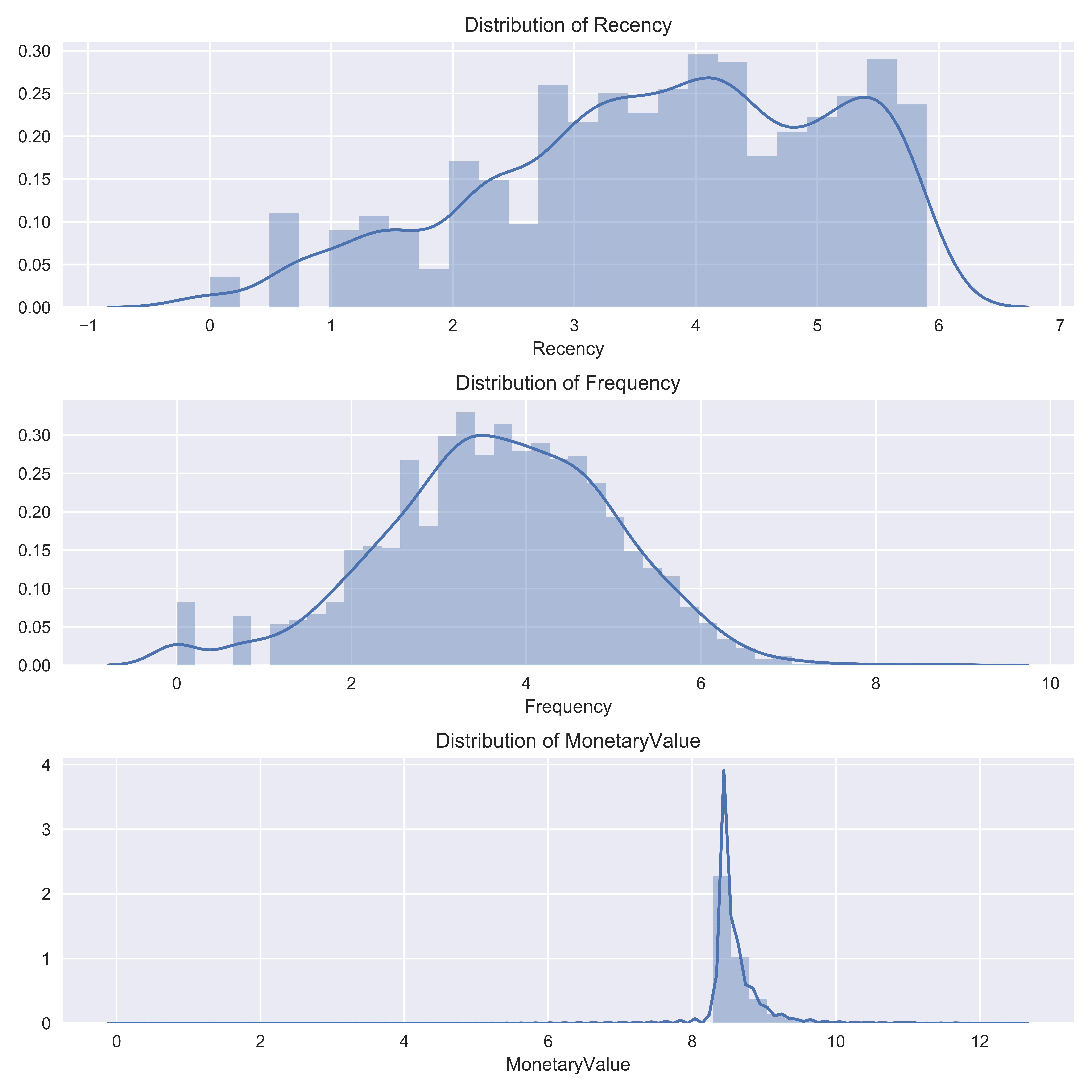Distribution Plots of RFM. The skewness is reduced after log transformation.

Once the skewness is reduced, I standardised the data by centering and scaling. Note all the variables now have a mean of 0 and a standard deviation of 1.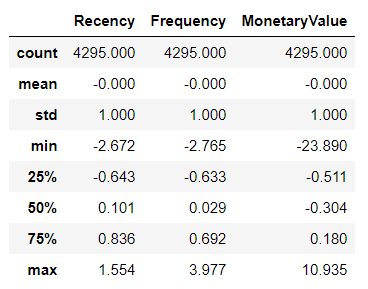Basic statistics of RFM. All variables have mean of 0 and standard deviation of 1 after centring and scaling.

## How about finding the optimal number of groups?

Once the data is prepared, the next step is to run iterations of K-means (usually up to 10 clusters) to calculate the Silhouette Score for each cluster.

``````def optimal_kmeans(dataset, start=2, end=11):
'''
Calculate the optimal number of kmeans

INPUT:
dataset : dataframe. Dataset for k-means to fit
start : int. Starting range of kmeans to test
end : int. Ending range of kmeans to test
OUTPUT:
Values and line plot of Silhouette Score.
'''

# Create empty lists to store values for plotting graphs
n_clu = []
km_ss = []

# Create a for loop to find optimal n_clusters
for n_clusters in range(start, end):

# Create cluster labels
kmeans = KMeans(n_clusters=n_clusters)
labels = kmeans.fit_predict(dataset)

# Calcualte model performance
silhouette_avg = round(silhouette_score(dataset, labels, random_state=1), 3)

# Append score to lists
km_ss.append(silhouette_avg)
n_clu.append(n_clusters)

print("No. Clusters: {}, Silhouette Score: {}, Change from Previous Cluster: {}".format(
n_clusters,
silhouette_avg,
(km_ss[n_clusters - start] - km_ss[n_clusters - start - 1]).round(3)))

# Plot graph at the end of loop
if n_clusters == end - 1:
plt.figure(figsize=(6.47,3))

plt.title('Silhouette Score')
sns.pointplot(x=n_clu, y=km_ss)
plt.savefig('silhouette_score.png', format='png', dpi=1000)
plt.tight_layout()
plt.show()``````

A lower Silhouette Score denotes the formation of better and more meaningful clusters; the result below shows the optimal number of clusters is four.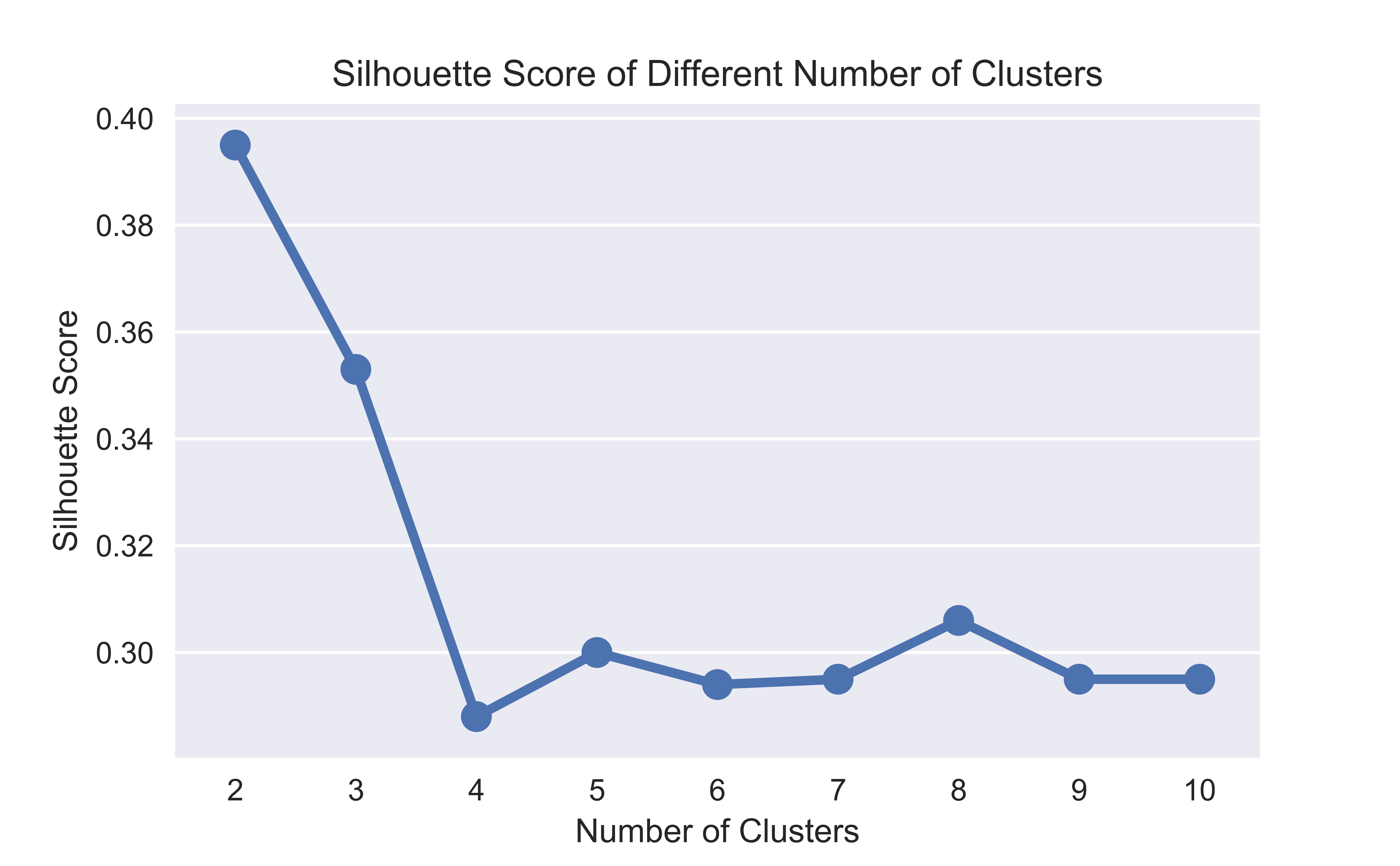Silhouette Score of 2 to 10 clusters. The optimal number of clusters is 4.

Nonetheless, it is a common practice to implement K-means clustering on +/- 1 of optimal cluster identified; here, it is 3, 4, and 5 clusters.

This gives a wider perspective and facilitates meaningful discussion with your stakeholders to determine the appropriate number of customer segments.

Perhaps there could be some market peculiarities and your stakeholders might decide to implement their marketing strategies on 5 clusters instead of the optimal 4 clusters identified.

## How does the end result of K-means segmentation look like?

Now we are ready to run the data through K-means of 3, 4 and 5 clusters to segment our customers.

``````def kmeans(df, clusters_number):
'''
Implement k-means clustering on dataset

INPUT:
dataset : dataframe. Dataset for k-means to fit.
clusters_number : int. Number of clusters to form.
end : int. Ending range of kmeans to test.
OUTPUT:
Cluster results and t-SNE visualisation of clusters.
'''

kmeans = KMeans(n_clusters = clusters_number, random_state = 1)
kmeans.fit(df)

# Extract cluster labels
cluster_labels = kmeans.labels_

# Create a cluster label column in original dataset
df_new = df.assign(Cluster = cluster_labels)

# Initialise TSNE
model = TSNE(random_state=1)
transformed = model.fit_transform(df)

# Plot t-SNE
plt.title('Flattened Graph of {} Clusters'.format(clusters_number))
sns.scatterplot(x=transformed[:,0], y=transformed[:,1], hue=cluster_labels, style=cluster_labels, palette="Set1")

return df_new, cluster_labels``````

Below is the result of the customer segmentation: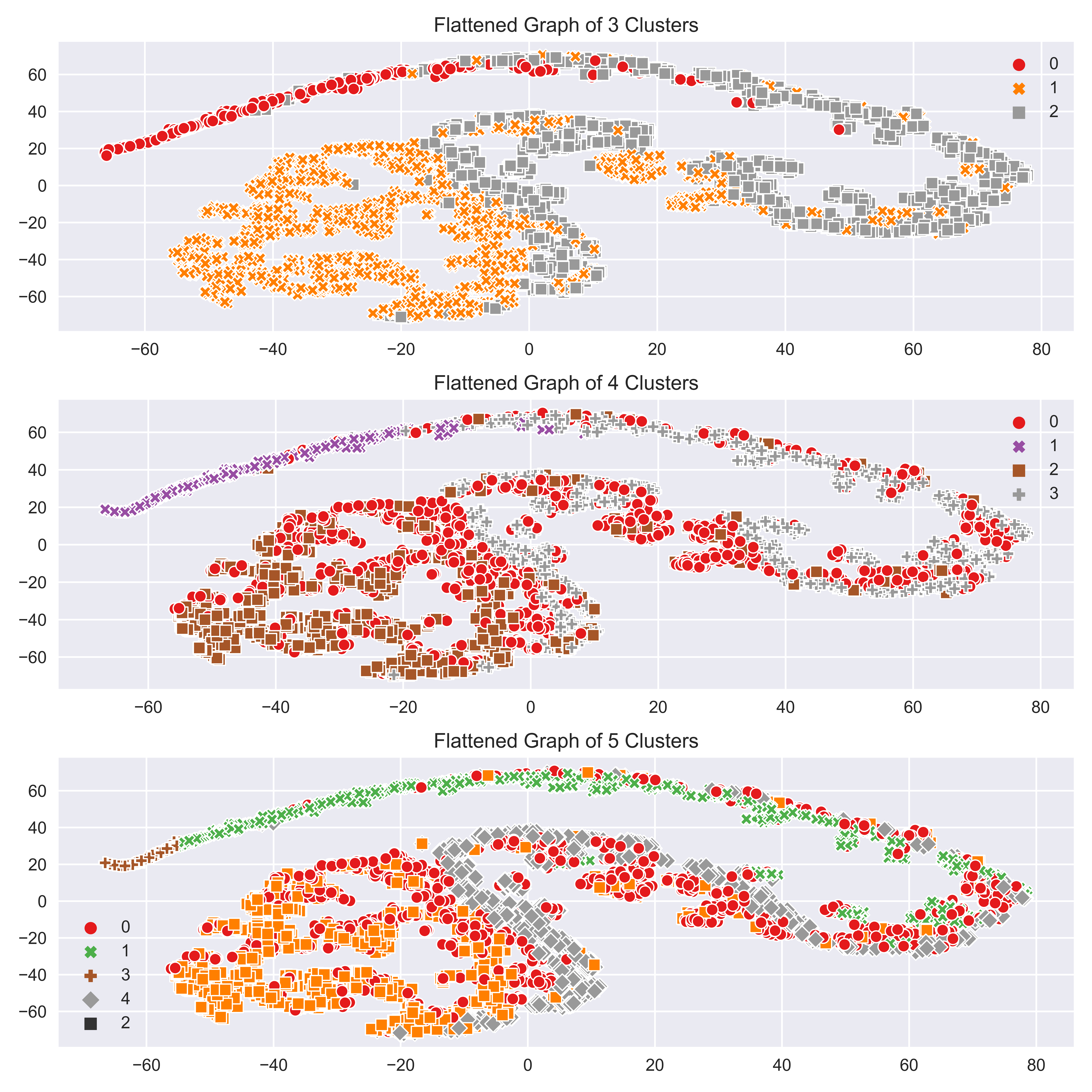Flattened (t-SNE) graph of 3,4 and5 clusters.

Recall that each information associated with a customer creates an additional dimension. The above image is obtained by flattening three-dimensional graphs (created from Recency, Frequency, and MonetaryValue) into two-dimensional graphs for ease of visualisation.

This visualisation can give you a sense of how well the clusters are formed.

In case you are wondering, the technique for flattening high dimensional graph and visualising it in a two-dimensional format is known as t-Distributed Stochastic Neighbor Embedding (t-SNE). You can read up more on this if you are interested; the explanation for this is beyond the scope of this article.

## How do I make use of the segmentation results in my marketing?

By this stage, each customer in the dataset has been tagged with their respective group number. You can proceed to use any industry common practice to visualise the results.

Below is an example of using Snake Plot and Relative Importance of Attributes Chart to build personas of each cluster of the segmentation. Both are commonly used in the marketing industry for customer segmentation.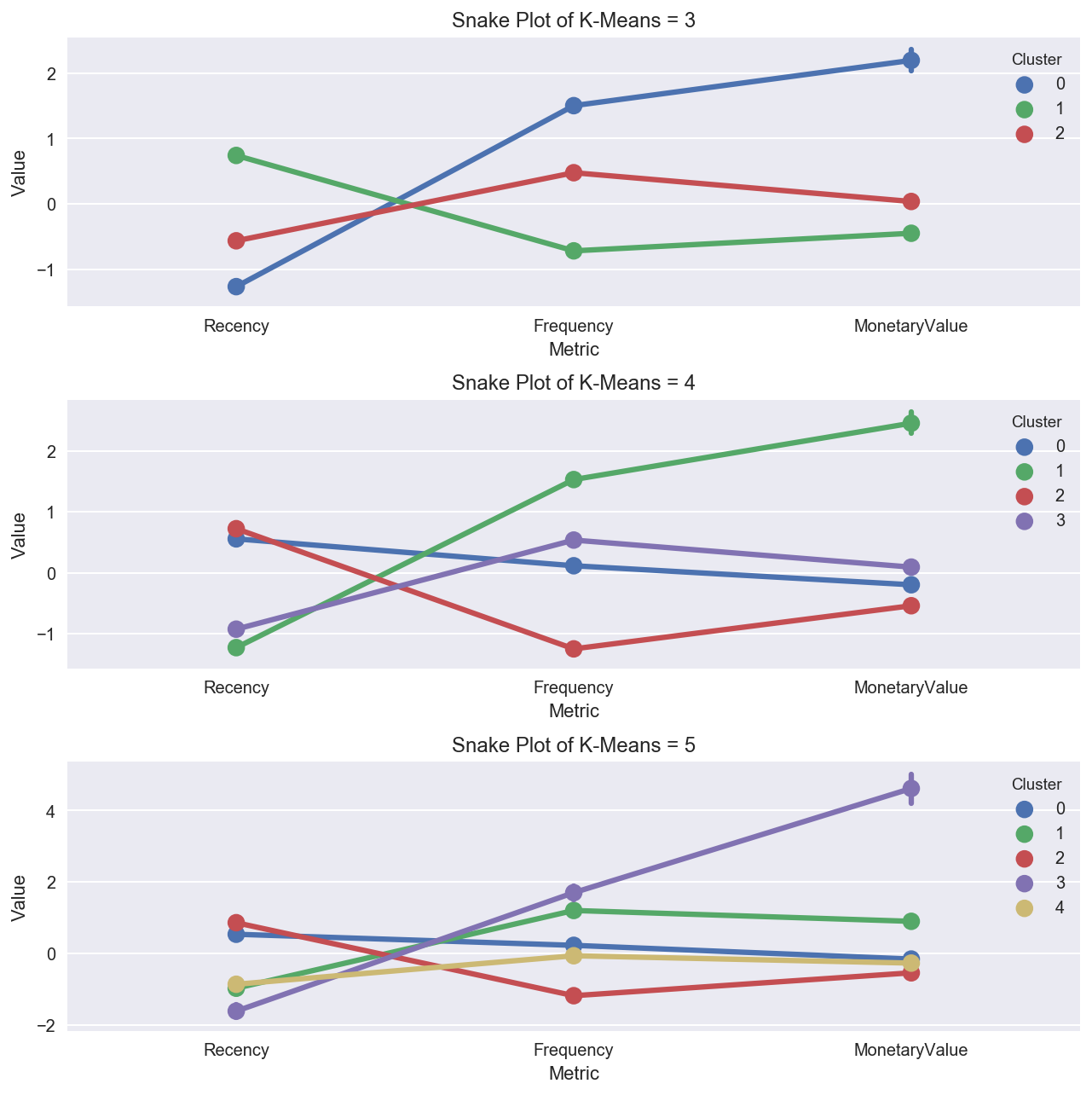Snake Plot of 3, 4, 5 clusters formed using K-means.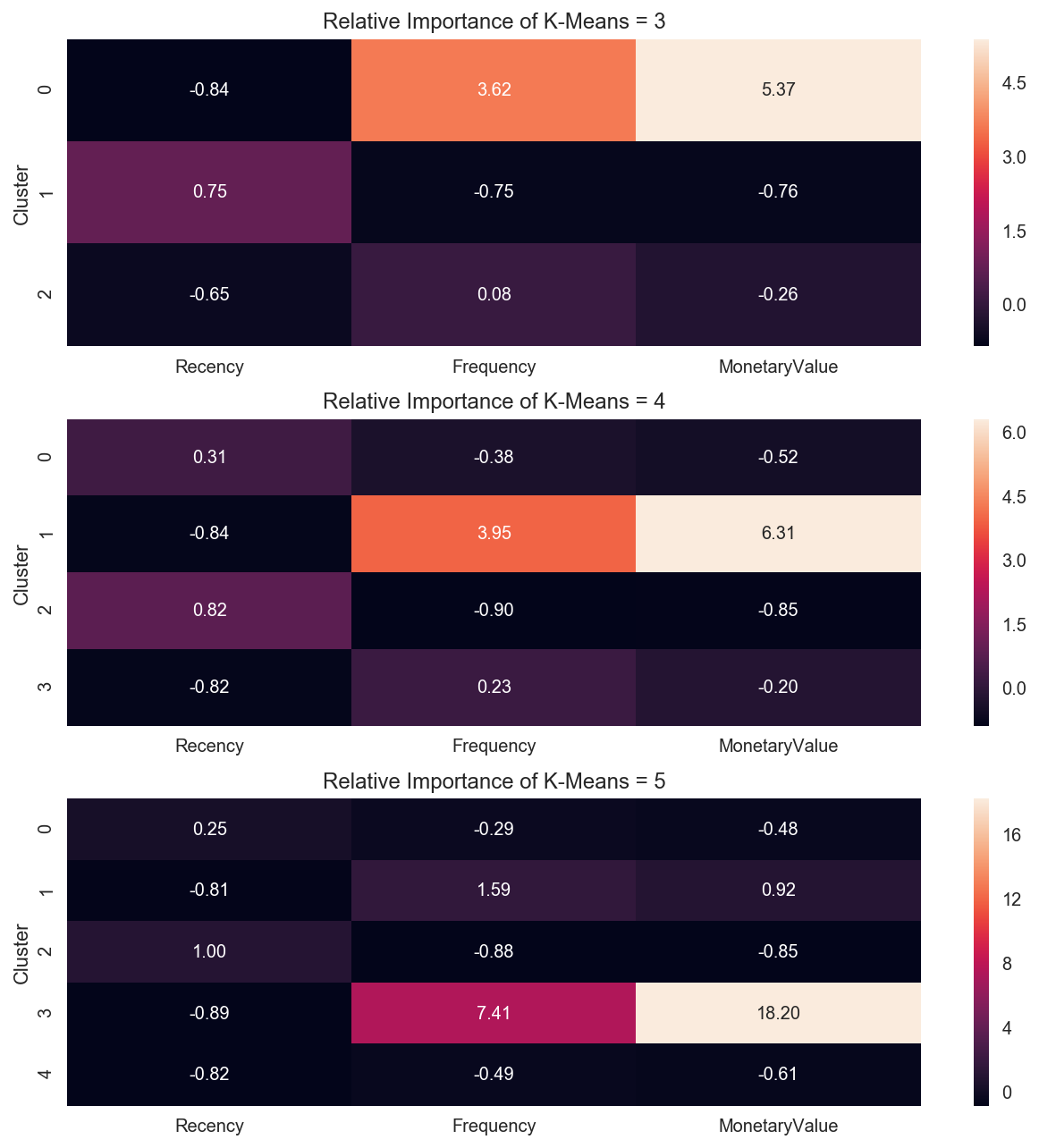Relative Importance Chart of 3, 4, and 5 clusters formed using K-means.

You can take this result and compare it against your original segmentation done using traditional methods. Is there any big difference?

It is a good practice to perform a deep dive and understand why K-means thinks customers of a particular group belong together (yes, sadly K-means is unable to write us a marketing report on their segmentation decision yet).

With this understanding, you could initiate discussion with relevant stakeholders to seek their opinion and get alignment on how to best segment the customers before launching the next big marketing campaign.

All the relevant codes for this article can be found at my repo.

# Conclusion

K-means is a simple but powerful segmentation method. Anyone doing customer or market segmentation should use this to augment traditional methods. Otherwise, they risk becoming obsolete in the age of artificial intelligence.

If you are keen to learn more about Unsupervised Learning and Clustering Methods, AISG has a course for it.

## Author

•Tern Poh is an Assistant Head at AI Singapore. He provides consulting services to enable customers to undertake the development and implementation of AI minimum viable models within their organisations.